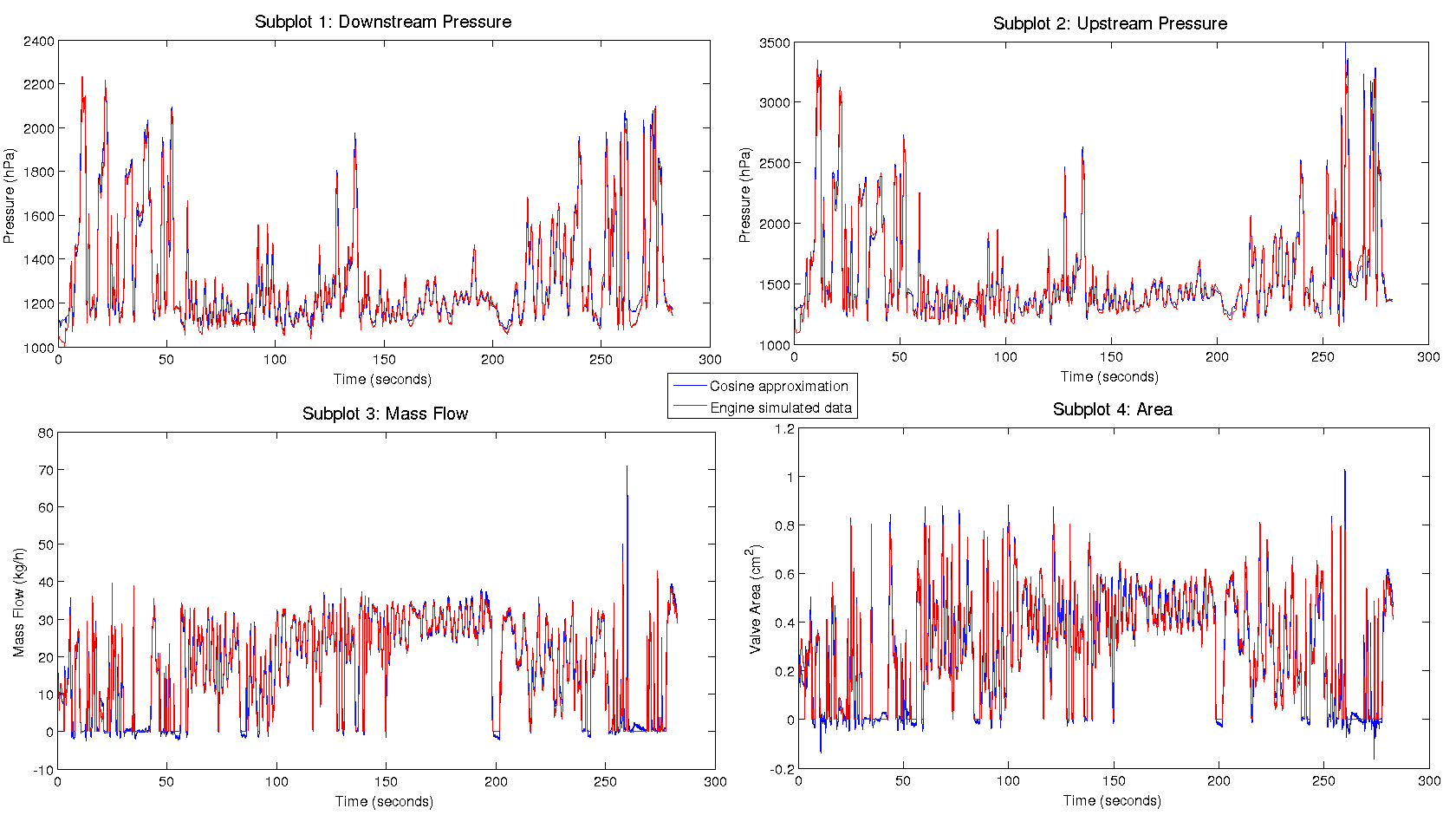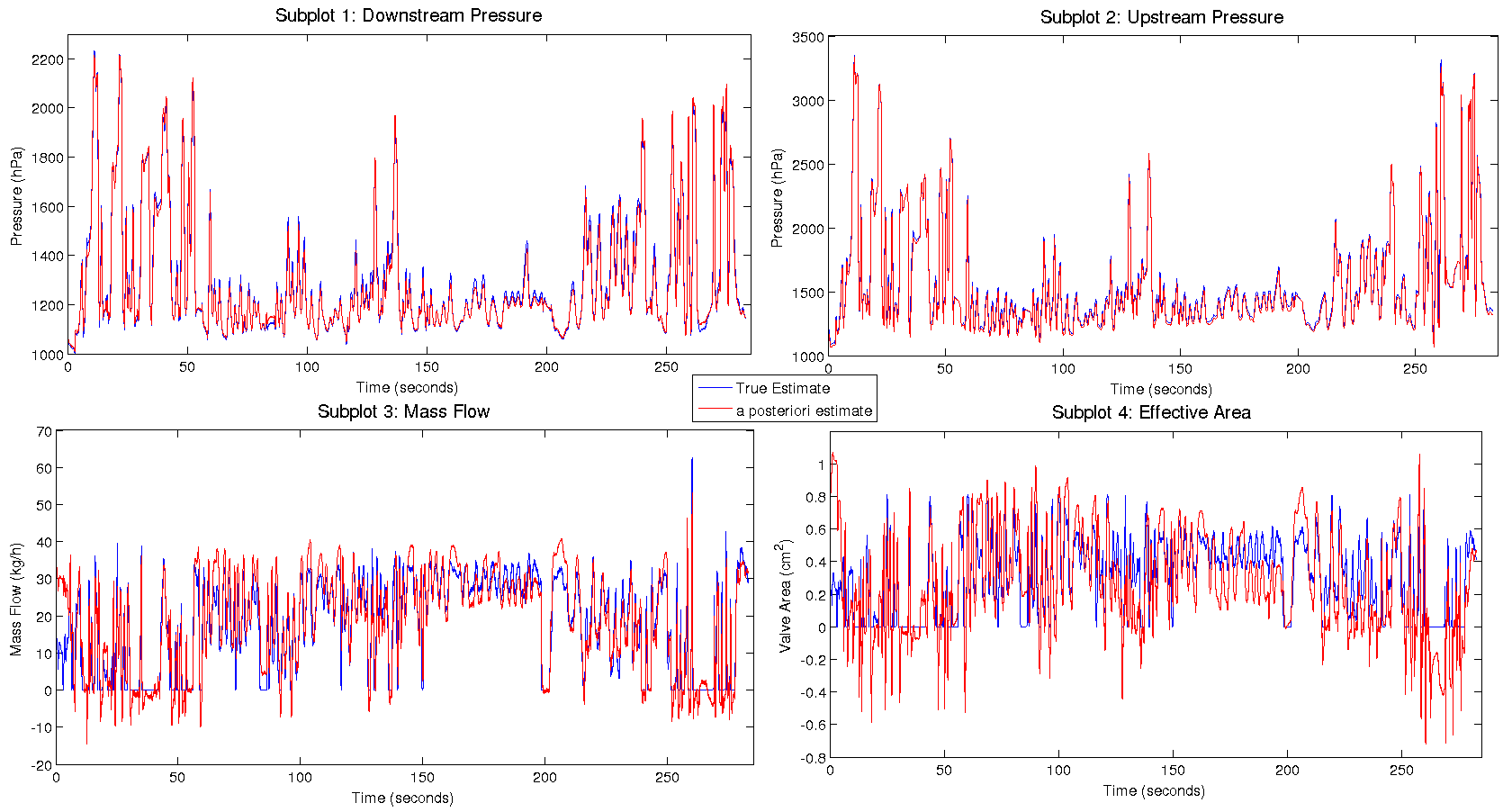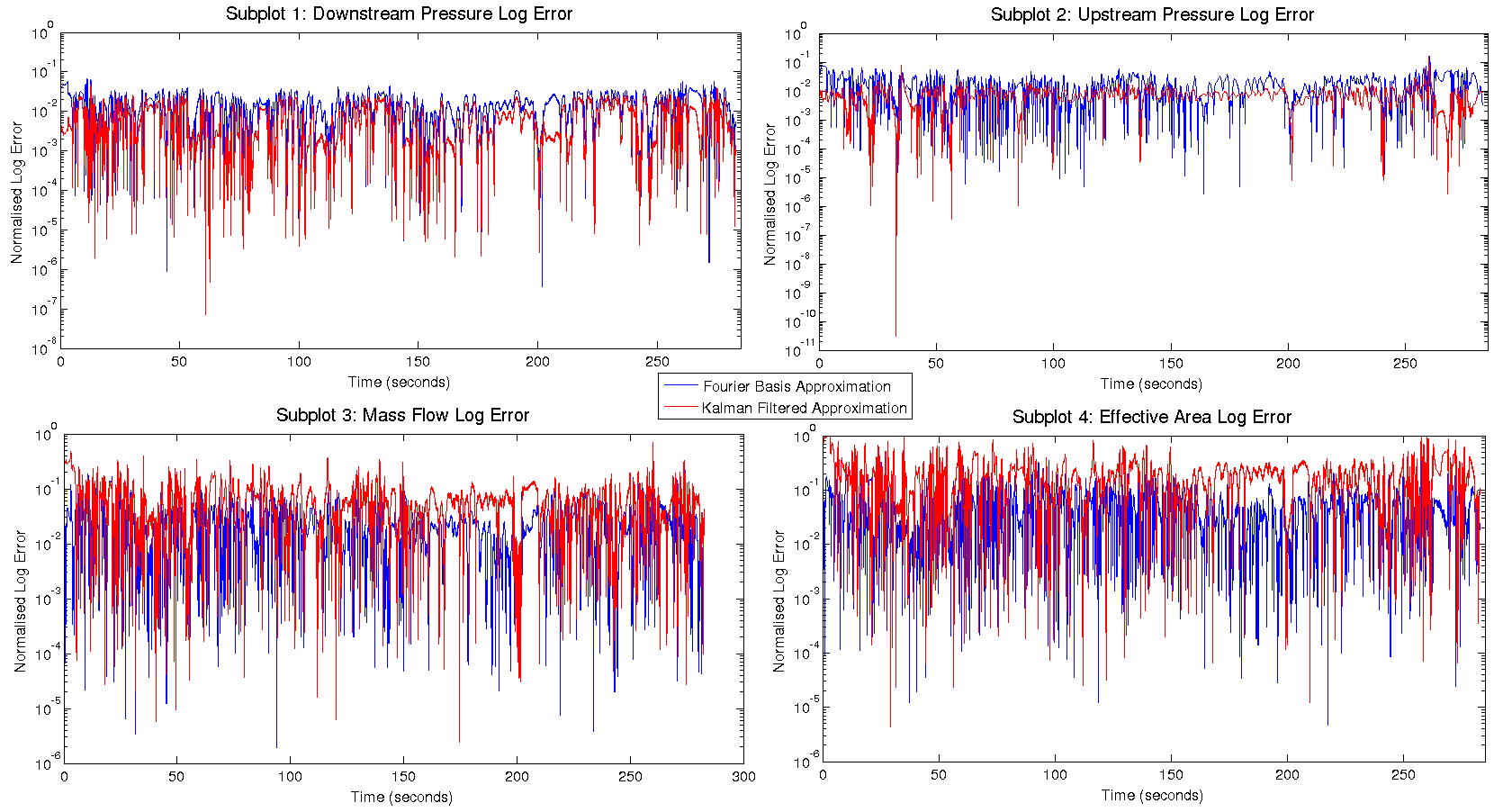# Numerical Results

### Fourier Space Approximation

We implemented a Fourier approximation as in the Least Squares page and the results are the graph as follows. To the naked eye, this approximation looks good, except negative areas are predicted, which is unphysical. A log error plot is shown below in the comparison section to show more explicitly how good it is.### Kalman Filtering Approximation

An extended Kalman filter was implemented so as to use this Fourier approximation as well as input data gathered. As can be seen below, this is effective with the pressure plots, and looks successful with the mass flow, but the effective area is a poor fit. The last point is due to the fact that the Fourier approximation and the input data for the Kalman filter area values do not agree, and the data is a shift down of the approximation, and so the two combine much lower than the true estimate.### Comparison

We now plot the normalised error in a logarithmic plot to see how good each approximation was. As one can see, the Kalman filtered method produces much better estimates for the upstream and downstream pressures, but for valve area and mass flow it is somewhat worse. This may well be due to the input data being particularly poor.